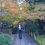# A modified Ramanujan–Nagell equation

In the field of number theory, the Ramanujan–Nagell equation is an equation between a square number and a number that is seven less than a power of two. It is an example of an exponential Diophantine equation, an equation to be solved in integers where one of the variables appears as an exponent.

It is named after Srinivasa Ramanujan, who conjectured that it has only five integer solutions, and after Trygve Nagell, who proved the conjecture.

The equation is

2^n - 7 = x^2

and solutions in natural numbers n and x exist just when n = 3, 4, 5, 7 and 15.

This was conjectured in 1913 by Indian mathematician Srinivasa Ramanujan, proposed independently in 1943 by the Norwegian mathematician Wilhelm Ljunggren, and proved in 1948 by the Norwegian mathematician Trygve Nagell.

The values of n correspond to the values of x as:- n = 3, 4, 5, 7 and 15 x = 1, 3, 5, 11 and 181.

Similarly what can we say about the equation:

2^n - 3 = x^3 ?

How many solutions does it have ?

I can only think of 2 solutions : ( n = 2 and x = 1 ) and (n = 7 = x = 5)

How can we prove that there are only two solutions to the above equation ?

Can any one come up with a 3rd solution ?Note by Vijay Simha
5 years, 7 months ago

This discussion board is a place to discuss our Daily Challenges and the math and science related to those challenges. Explanations are more than just a solution — they should explain the steps and thinking strategies that you used to obtain the solution. Comments should further the discussion of math and science.

When posting on Brilliant:

• Use the emojis to react to an explanation, whether you're congratulating a job well done , or just really confused .
• Ask specific questions about the challenge or the steps in somebody's explanation. Well-posed questions can add a lot to the discussion, but posting "I don't understand!" doesn't help anyone.
• Try to contribute something new to the discussion, whether it is an extension, generalization or other idea related to the challenge.
• Stay on topic — we're all here to learn more about math and science, not to hear about your favorite get-rich-quick scheme or current world events.

MarkdownAppears as
*italics* or _italics_ italics
**bold** or __bold__ bold
- bulleted- list
• bulleted
• list
1. numbered2. list
1. numbered
2. list
Note: you must add a full line of space before and after lists for them to show up correctly
paragraph 1paragraph 2

paragraph 1

paragraph 2

[example link](https://brilliant.org)example link
> This is a quote
This is a quote
    # I indented these lines
# 4 spaces, and now they show
# up as a code block.

print "hello world"
# I indented these lines
# 4 spaces, and now they show
# up as a code block.

print "hello world"
MathAppears as
Remember to wrap math in $$ ... $$ or $ ... $ to ensure proper formatting.
2 \times 3 $2 \times 3$
2^{34} $2^{34}$
a_{i-1} $a_{i-1}$
\frac{2}{3} $\frac{2}{3}$
\sqrt{2} $\sqrt{2}$
\sum_{i=1}^3 $\sum_{i=1}^3$
\sin \theta $\sin \theta$
\boxed{123} $\boxed{123}$

## Comments

Sort by:

Top Newest

Thanks for explaining.

- 1 year, 4 months ago

Log in to reply

It looks like having no further solution. But I am not enough strong to prove that. U can think of programming this out. Then for large value u will have searches.

- 5 years, 6 months ago

Log in to reply

×

Problem Loading...

Note Loading...

Set Loading...